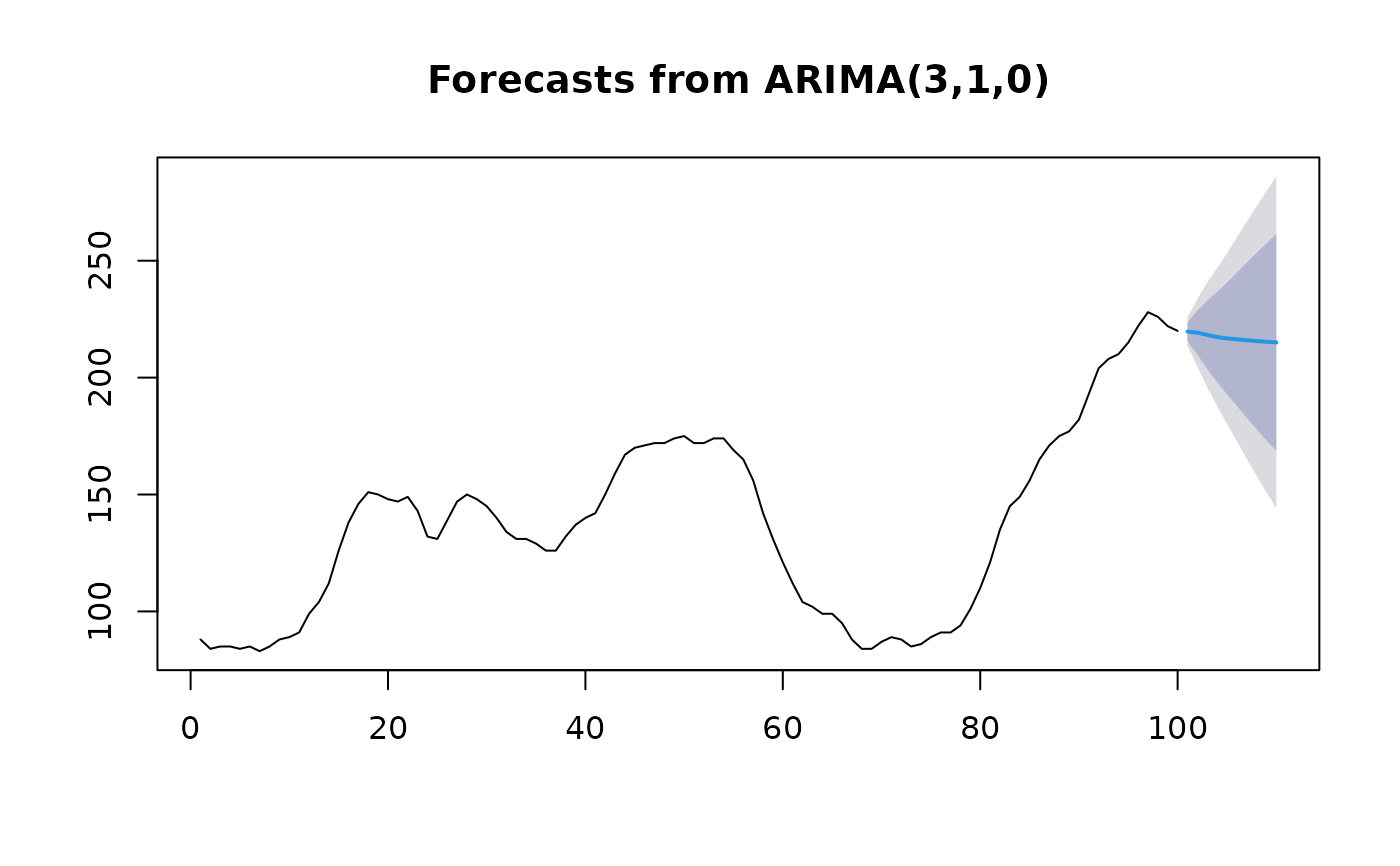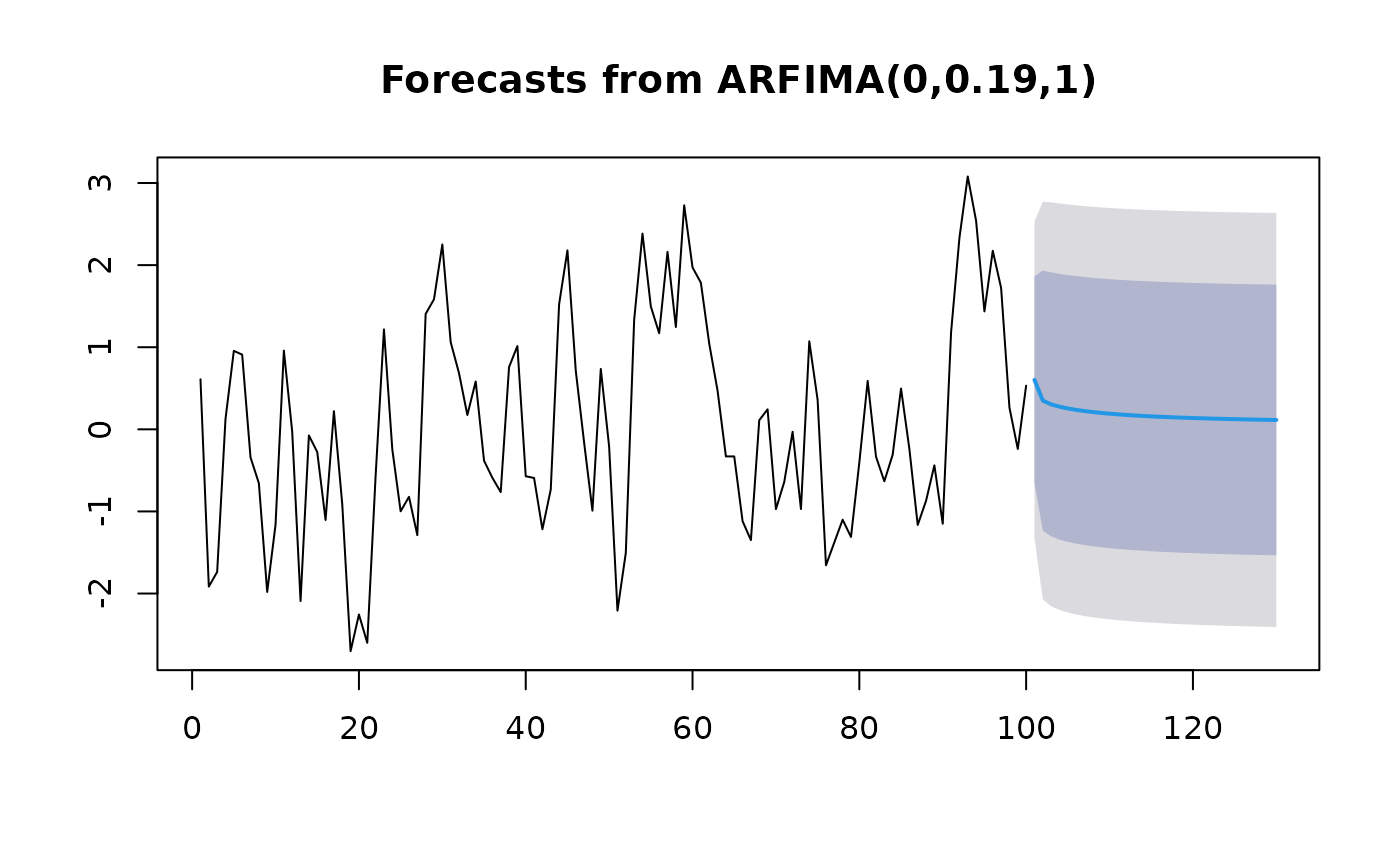Returns forecasts and other information for univariate ARIMA models.

# S3 method for fracdiff
forecast(
object,
h = 10,
level = c(80, 95),
fan = FALSE,
lambda = object$lambda, biasadj = NULL, ... ) # S3 method for Arima forecast( object, h = ifelse(object$arma > 1, 2 * object$arma, 10), level = c(80, 95), fan = FALSE, xreg = NULL, lambda = object$lambda,
bootstrap = FALSE,
npaths = 5000,
...
)

# S3 method for ar
forecast(
object,
h = 10,
level = c(80, 95),
fan = FALSE,
lambda = NULL,
bootstrap = FALSE,
npaths = 5000,
...
)

## Arguments

object

An object of class "Arima", "ar" or "fracdiff". Usually the result of a call to arima, auto.arima, ar, arfima or fracdiff.

h

Number of periods for forecasting. If xreg is used, h is ignored and the number of forecast periods is set to the number of rows of xreg.

level

Confidence level for prediction intervals.

fan

If TRUE, level is set to seq(51,99,by=3). This is suitable for fan plots.

lambda

Box-Cox transformation parameter. If lambda="auto", then a transformation is automatically selected using BoxCox.lambda. The transformation is ignored if NULL. Otherwise, data transformed before model is estimated.

Use adjusted back-transformed mean for Box-Cox transformations. If transformed data is used to produce forecasts and fitted values, a regular back transformation will result in median forecasts. If biasadj is TRUE, an adjustment will be made to produce mean forecasts and fitted values.

...

Other arguments.

xreg

Future values of an regression variables (for class Arima objects only). A numerical vector or matrix of external regressors; it should not be a data frame.

bootstrap

If TRUE, then prediction intervals computed using simulation with resampled errors.

npaths

Number of sample paths used in computing simulated prediction intervals when bootstrap=TRUE.

## Value

An object of class "forecast".

The function summary is used to obtain and print a summary of the results, while the function plot produces a plot of the forecasts and prediction intervals.

The generic accessor functions fitted.values and residuals

extract useful features of the value returned by forecast.Arima.

An object of class "forecast" is a list containing at least the following elements:

model

A list containing information about the fitted model

method

The name of the forecasting method as a character string

mean

Point forecasts as a time series

lower

Lower limits for prediction intervals

upper

Upper limits for prediction intervals

level

The confidence values associated with the prediction intervals

x

The original time series (either object itself or the time series used to create the model stored as object).

residuals

Residuals from the fitted model. That is x minus fitted values.

fitted

Fitted values (one-step forecasts)

## Details

For Arima or ar objects, the function calls predict.Arima or predict.ar and constructs an object of class "forecast" from the results. For fracdiff objects, the calculations are all done within forecast.fracdiff using the equations given by Peiris and Perera (1988).

predict.Arima, predict.ar, auto.arima, Arima, arima, ar, arfima.

Rob J Hyndman

## Examples

fit <- Arima(WWWusage,c(3,1,0))
plot(forecast(fit))library(fracdiff)
x <- fracdiff.sim( 100, ma=-.4, d=.3)\$series
fit <- arfima(x)
plot(forecast(fit,h=30))